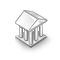Interesting script?
So post a link to it -
let others appraise it
You liked the script? Try it in the MetaTrader 5 terminal# GRNN Neural Network Class - library for MetaTrader 5

Views:
6209
Rating:
Published:
2012.12.25 11:31

The CNetGRNN class realizes a generalized regression neural network (General Regression Neural Network - GRNN).

Creation of the network is declared to be the class parametric constructor.

`CNetGRNN *net=new CNetGRNN(input vector size, output vector size);`

Learning network is provided by calling the Learn method (the number of learning patterns, input data array, output data array, the number of learning cycles, the maximum learning error).

Input and output learning data are located in one-dimensional arrays vector by vector. The learning process is limited either by the number of learning epochs or admissible error.

The Learn method returns the following values:

• 0 - network learning is completed and learning result can be checked through the class variable: mse – learning error, epoch – number of accomplished learning cycles;
• -4 - not enough memory.

The Calculate method (input vector array, network response array) is used to get response network.

Save (open file handle with FILE_WRITE and FILE_BIN flags) and Load (open file handle with FILE_READ and FILE_BIN flags) methods are intended for saving the network to a file and loading the network from the file respectively. Network topology, learning errors and array weights are saved to the file. If parameters of the loaded network topology differ from the parameters of the established network topology, the network will not be loaded and the Load method will return false.

Using of the class is shown in the attached specimens: Test_GRNN_XOR - learning network function "excluding OR", Test_GRNN_MUL_ADD - learning network multiplication and addition of integers.

Translated from Russian by MetaQuotes Software Corp.
Original code: https://www.mql5.com/ru/code/1324PNN Neural Network Class

The class realizes the probabilistic neural network (Probabilistic Neural Network - PNN)ATR_MA_Oscillator

ATR MA Oscillator is the oscillator drawn on the basis of the difference between the ATR oscillator and its signal line.iSarX4

Four parabolic indicators at one time!Aggressiveness

The Aggressiveness indicator displays how many points the instrument runs on the average for one candlestick